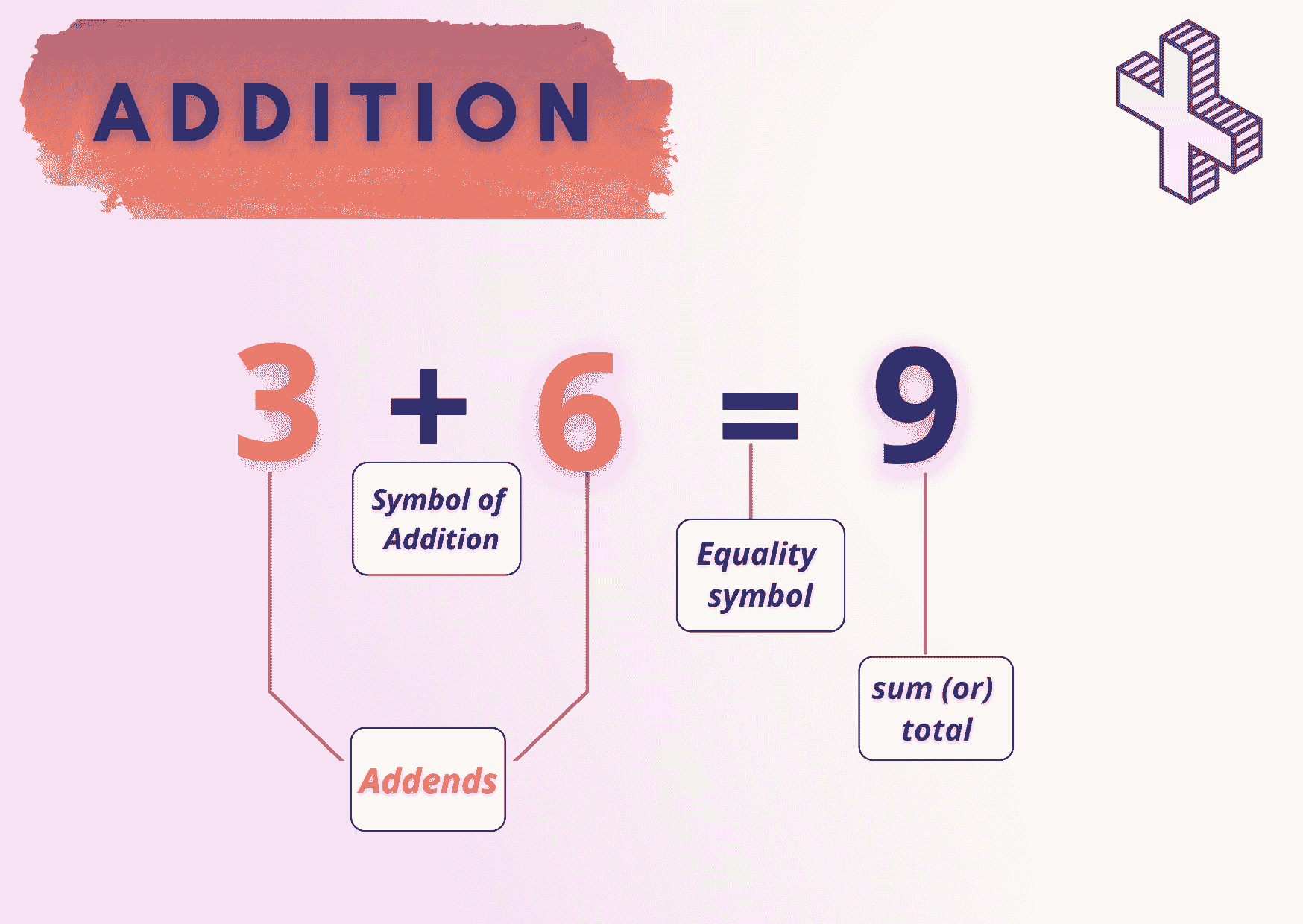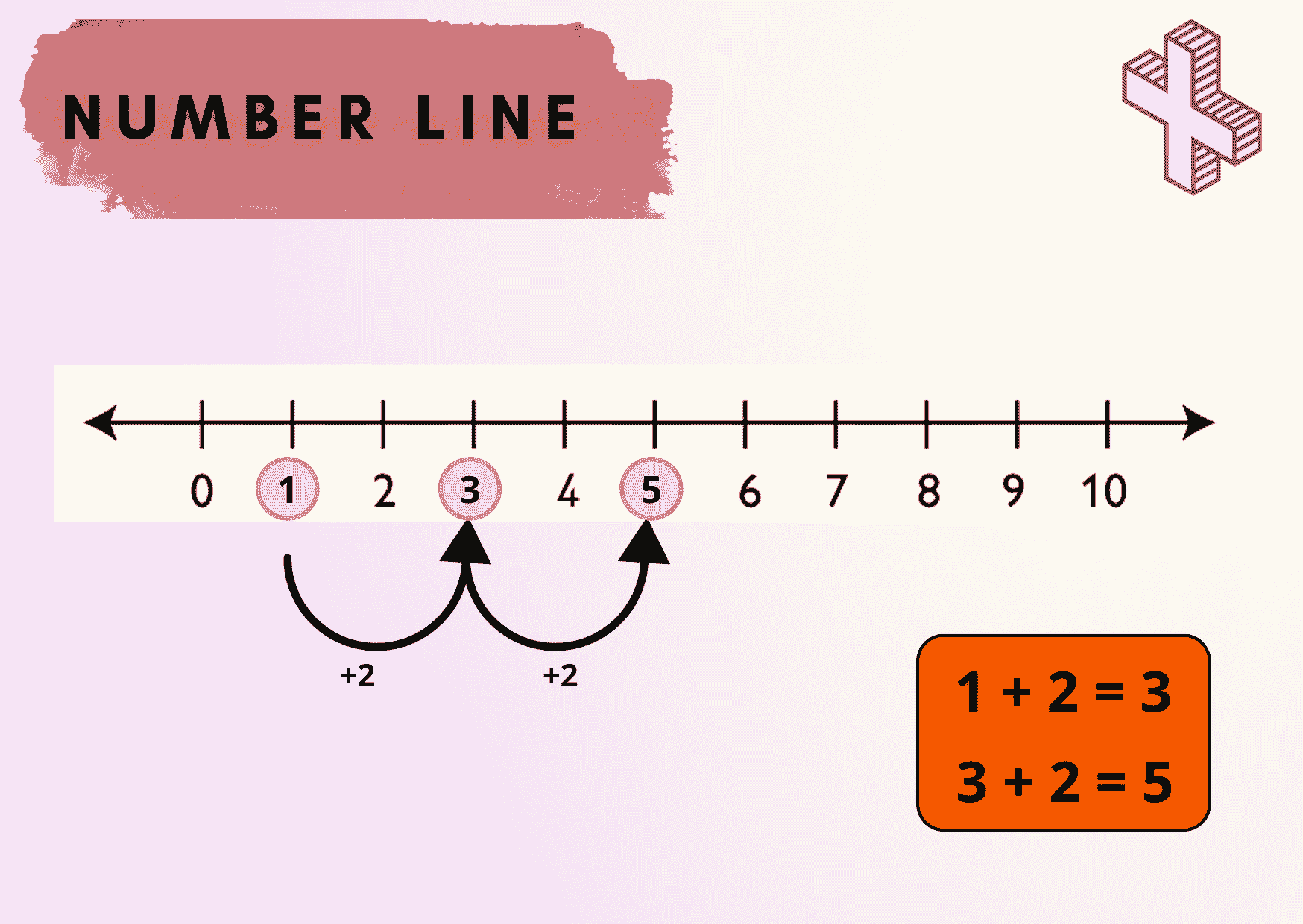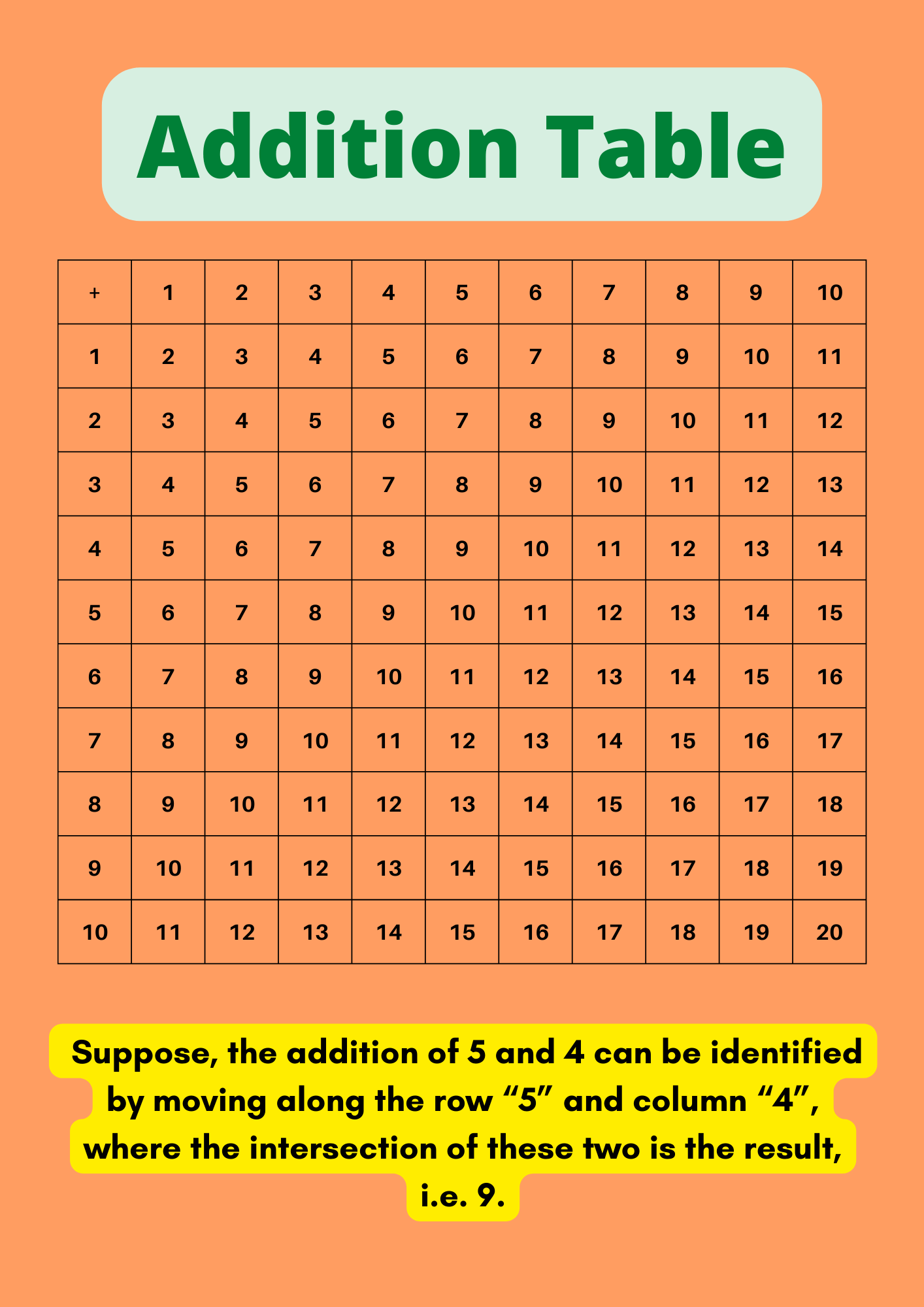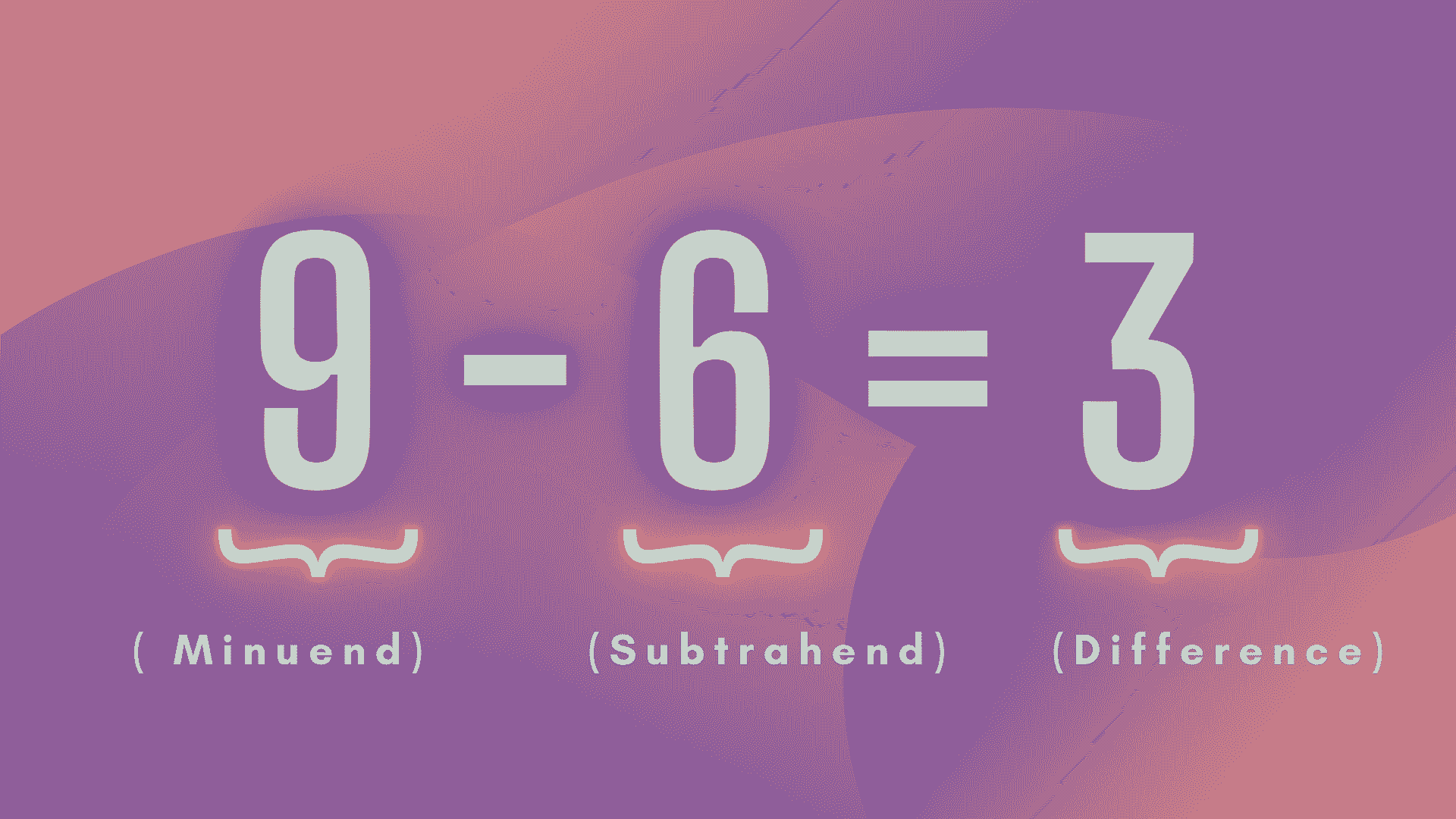Courses
Courses for Kids
Free study material
Free LIVE classes
More

# Addition and Subtraction in Year 5LIVE
Join Vedantu’s FREE Mastercalss

## Becoming Fluent in Addition and Subtraction

Every day, we face a variety of situations in which we do simple arithmetic operations. We are already familiar with numbers and the number system. Now we will discuss two simple arithmetic operations, that is, addition and subtraction.

These are two important arithmetic operations where we add and subtract two or more numbers or mathematical values. The symbol that represents addition is ‘+’ and subtraction is ‘-’.

One of the most simple topics for school kids is addition of numbers.The term "addition" means the process of adding two or more numbers. The plus sign '+' is used to signify addition. For example, the addition of 3 and 6 can be written as 3 + 6.

## Addition Properties for Year 5 Kids

The addition of two or more numbers has some important properties. We’ll discuss list of those properties of addition below:

• When two whole numbers are added together, the result is another whole number.

• When two natural numbers are added together, the result is another natural number.

• Two integers added together will give an integer; two positive integers added together will be a positive integer; two negative integers added together will be a negative number; and one positive and one negative integer added together will be an integer with the same sign which the largest number has.

• When two rational numbers are added together, the result is a rational number once again. Also, the properties relating to positive and negative signs will be the same as they are for integers.

• Any number added to 0 equals the number itself.

There are different methods to add numbers. They are:

• By counting figures: Here we’ll just start counting the figures from 1.

• With the help of a number line: On a number line, addition is as simple as counting positive numbers as you move towards the right-hand side. It helps you in imagining the addition operation with small numbers.• With regrouping: When adding two or more numbers of any size, addition with regrouping is a method used. It's applied with the column method of addition, which arranges sums vertically and column numbers are added one at a time.

• With the help of addition tables: The addition table chart from numbers 1 to 10 is shown in the figure below.## Subtraction for Year 5

The operation of removing objects from a collection is done by subtraction. Subtraction is symbolised by the minus sign. If there are 8 apples arranged in a basket, and 4 apples are taken out, there will be 8 – 4 apples left in the basket. So , the difference between 8 and 4 is 4, or 8 - 4 = 4.Parts of a Subtraction Problem

## Subtraction Properties for Year 5 Kids

Subtraction of numbers possess some properties as given below:

• Subtraction of 0 from any number gives the number itself, implying that the difference is nil.

• When we subtract 1 from any number, the difference always equals the number's predecessor.

• Number subtraction is anti-commutative.

• Subtraction of numbers is a non-associative operation.

## Solved Examples

1. What is the sum of 5071 and 3412?

Solution: Sum is nothing but the total of two given numbers.

⇒ 5071+3412= 8483

Hence, the sum of 5071 and 3412 is 8483.

2. What is the difference between 8731 and 3128?

Solution: Difference is nothing but subtraction.

⇒ 8731-3128= 5603

Hence, the difference between 8731 and 3128 is 5603.

3. How much smaller is 3512 than 9291?

Solution: To find the solution, we have to find the difference between 3512 and 9291.

So, we know that difference is nothing but subtraction.

⇒ 9291-3512= 5779

Therefore, 3512 is smaller as it is 5779 less.

4. Which is greater, 4929 or 9129 and by how much?

Solution: By looking at the numbers we can easily say that 9129 is greater than 4929.

To find how much greater, we have to find the difference between 4929 and 9129.

⇒ 9129-4929= 4200

Therefore, 9129 is bigger as it is 4200 more than 4929.

## Conclusion

The progress of a strong understanding of addition and subtraction is required for the development of later concepts such as other arithmetic operations, measurements-based calculations, and algebra. A kid can learn the fundamentals of addition while studying the number system.

Last updated date: 30th Sep 2023
Total views: 122.7k
Views today: 3.22k

## FAQs on Addition and Subtraction in Year 5

1. How many types of addition methods are there?

There are different methods to perform addition:

• By using a number line